Open Access Publications from the University of California

## Recent Work

UC Davis is situated in the heart of California. Founded in 1933, the Department of Mathematics stays relevant in mathmatics research both through continuing research and in discovering new talent. Research is encouraged across all levels, from undergraduate through graduate, as well as through outreach programs.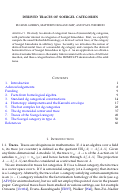## Derived Traces of Soergel Categories

(2022)

We study two kinds of categorical traces of (monoidal) dg categories, with particular interest in categories of Soergel bimodules. First, we explicitly compute the usual Hochschild homology, or derived vertical trace, of the category of Soergel bimodules in arbitrary types. Secondly, we introduce the notion of derived horizontal trace of a monoidal dg category and compute the derived horizontal trace of Soergel bimodules in type A. As an application we obtain a derived annular Khovanov-Rozansky link invariant with an action of full twist insertion, and thus a categorification of the HOMFLY-PT skein module of the solid torus.

## eGHWT: The Extended Generalized Haar–Walsh Transform

(2022)

Extending computational harmonic analysis tools from the classical setting of regular lattices to the more general setting of graphs and networks is very important, and much research has been done recently. The generalized Haar–Walsh transform (GHWT) developed by Irion and Saito (2014) is a multiscale transform for signals on graphs, which is a generalization of the classical Haar and Walsh–Hadamard transforms. We propose the extended generalized Haar–Walsh transform (eGHWT), which is a generalization of the adapted time–frequency tilings of Thiele and Villemoes (1996). The eGHWT examines not only the efficiency of graph-domain partitions but also that of “sequency-domain” partitions simultaneously. Consequently, the eGHWT and its associated best-basis selection algorithm for graph signals significantly improve the performance of the previous GHWT with the similar computational cost, O(Nlog N) , where N is the number of nodes of an input graph. While the GHWT best-basis algorithm seeks the most suitable orthonormal basis for a given task among more than (1.5) N possible orthonormal bases in RN, the eGHWT best-basis algorithm can find a better one by searching through more than 0.618 · (1.84) N possible orthonormal bases in RN. This article describes the details of the eGHWT best-basis algorithm and demonstrates its superiority using several examples including genuine graph signals as well as conventional digital images viewed as graph signals. Furthermore, we also show how the eGHWT can be extended to 2D signals and matrix-form data by viewing them as a tensor product of graphs generated from their columns and rows and demonstrate its effectiveness on applications such as image approximation.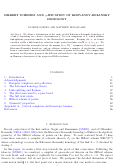## Hilbert schemes and y–ification of Khovanov–Rozansky homology

(2022)

We define a deformation of the triply graded Khovanov–Rozansky homology of a link L depending on a choice of parameters yc for each component of L, which satisfies link-splitting properties similar to the Batson–Seed invariant. Keeping the yc as formal variables yields a link homology valued in triply graded modules over ℚ[xc, yc]c∈π0(L). We conjecture that this invariant restores the missing Q ↔ T Q-1 symmetry of the triply graded Khovanov–Rozansky homology, and in addition satisfies a number of predictions coming from a conjectural connection with Hilbert schemes of points in the plane. We compute this invariant for all positive powers of the full twist and match it to the family of ideals appearing in Haiman’s description of the isospectral Hilbert schem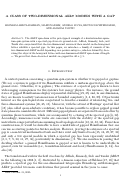## A class of two-dimensional AKLT models with a gap

(2021)

The AKLT spin chain is the prototypical example of a frustration-free quantum spin system with a spectral gap above its ground state. Affleck, Kennedy, Lieb, and Tasaki also conjectured that the two-dimensional version of their model on the hexagonal lattice exhibits a spectral gap. In this paper, we introduce a family of variants of the two-dimensional AKLT model depending on a positive integer n, which is defined by decorating the edges of the hexagonal lattice with one-dimensional AKLT spin chains of length $n$. We prove that these decorated models are gapped for all $n\geq 3$.

(2021)

## Natural Graph Wavelet Packet Dictionaries

(2021)

We introduce a set of novel multiscale basis transforms for signals on graphs that utilize their “dual” domains by incorporating the “natural” distances between graph Laplacian eigenvectors, rather than simply using the eigenvalue ordering. These basis dictionaries can be seen as generalizations of the classical Shannon wavelet packet dictionary to arbitrary graphs, and do not rely on the frequency interpretation of Laplacian eigenvalues. We describe the algorithms (involving either vector rotations or orthogonalizations) to construct these basis dictionaries, use them to efficiently approximate graph signals through the best basis search, and demonstrate the strengths of these basis dictionaries for graph signals measured on sunflower graphs and street networks.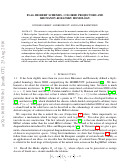## Flag Hilbert schemes, colored projectors and Khovanov-Rozansky homology

(2021)

We construct a categorification of the maximal commutative subalgebra of the type A Hecke algebra. Specifically, we propose a monoidal functor from the (symmetric) monoidal category of coherent sheaves on the flag Hilbert scheme to the (non-symmetric) monoidal category of Soergel bimodules. The adjoint of this functor allows one to match the Hochschild homology of any braid with the Euler characteristic of a sheaf on the flag Hilbert scheme. The categorified Jones-Wenzl projectors studied by Abel, Elias and Hogancamp are idempotents in the category of Soergel bimodules, and they correspond to the renormalized Koszul complexes of the torus fixed points on the flag Hilbert scheme. As a consequence, we conjecture that the endomorphism algebras of the categorified projectors correspond to the dg algebras of functions on affine charts of the flag Hilbert schemes. We define a family of differentials dN on these dg algebras and conjecture that their homology matches that of the glN projectors, generalizing earlier conjectures of the first and third authors with Oblomkov and Shende.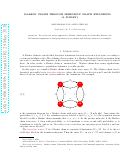## Markov Chains Through Semigroup Graph Expansions (A Survey)

(2021)

We review the recent approach to Markov chains using the Karnofksy–Rhodes and McCammond expansions in semigroup theory by the authors and illustrate them by two examples.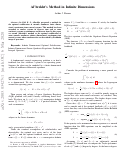## Al'brekht's Method in Infinite Dimensions

(2020)

In 1961 E. G. Albrekht presented a method for the optimal stabilization of smooth, nonlinear, finite dimensional, continuous time control systems. This method has been extended to similar systems in discrete time and to some stochastic systems in continuous and discrete time. In this paper we extend Albrekht's method to the optimal stabilization of some smooth, nonlinear, infinite dimensional, continuous time control systems whose nonlinearities are described by Fredholm integral operators.